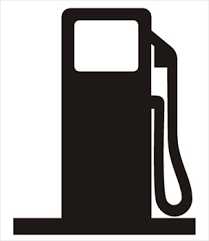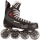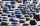# Diesel fuel

In the morning we refueled and set off. After the first stop we passed 20% of diesel, after the second 10% of the balance. At the third stop we had only 9 liters of diesel and we had to refuel. How big a tank do we have?

V =  12.5 l

### Step-by-step explanation:Did you find an error or inaccuracy? Feel free to write us. Thank you!Tips to related online calculators
Do you have a linear equation or system of equations and looking for its solution? Or do you have a quadratic equation?
Tip: Our volume units converter will help you with the conversion of volume units.

## Related math problems and questions:

• Solutions, mixturesWe have 2 liters of 20% solution available. How much 70% solution do we need to add to it to get a 30% solution?
• Mixture 2How many liters of water must be added to 7 liters of a 20% solution to obtain a 10% solution?
• FertilizerI have 4 liters of 10% fertilizer. How much water do I need to add to have a 2% solution?The business earned CZK 98,400 in the shop in three weeks. In the second week, sales were 20% higher than the first week. The third week was 10% lower than the second week. How much did business earn in each week?
• MerchantThe merchant lower cost by 10% in December when it was not sold. Again in January lower cost by 20% and now costs 576 €. A. How much the goods stood originally? B, how much cost the goods after the first lowering? C, How many percents totally merchant low
• GramsHow many grams of 5% solution do we need to add to 100g of 50% solution to get a 20% solution?
• Four numbersThe first number is 50% second, the second number is 40% third, the third number is 20% of the fourth. The sum is 396. What are the numbers?
• Alcohol solutionsWe have to produce 2 liters of 60% alcohol from 55% and 80%. How many of which ones will we use in the solution?
• Sales offThe price has decreased by 20%. How many percents do I have to raise the new price to be the same as before the cut?
• Sale offThe TV went down 10% and then 10% off the original price again. Now it costs 300 €. What was its original price?
• Car downSarah buys a car costing \$12,500. It depreciates value by 8% in the first year, 10% in the second year, and 5% in the third year. Calculate the value of a car after the third year.
• RewardThree workers shared a common reward 13110 CZK: the first worker got 35% less than the second, and the third worker got 20% more than the second worker. How much got each worker?
• RepairingThree employees earned a total of € 469 for repairing the equipment. They split so that the first got 20% more than the second, and the third 15% more than the second. How many euros did everyone get?
• Two shopsIn two different shops, the same skis had the same price. In the first, however, they first became more expensive by 20% and then cheaper by 5%. In the second, they were first cheaper by 5% and then more expensive by 30%, so after these adjustments in the
• Ice skatesIce skates were raised twice, the first time by 25%, the second time by 10%. After the second price, their cost was 82.5 euros. What was the original price of skates?
• Washing machineThe washing machine cost € 325. In sale-off, they became cheaper by 10%, later by another 10%. Find the last price of the washing machine.
• The dealerThe dealer planned to sell 40% of the cars he had in stock in May. However, he sold 20 less cars, ie only 15% of cars. a) How many cars did he have in stock? b) How many cars did he sell in May?